# Calculations Formula Examples

Calculations is a Pro Feature

Want some inspiration for using calculation formulas in your WordPress forms? With the WPForms Calculations addon, you can turn your forms into effective calculators, providing instant solutions for your users.

This guide will showcase various forms with example formulas to help spark your creativity.

Requirements:

• You’ll need the Pro license level or higher to access the Calculations addon
• Ensure that you’ve installed the latest version of WPForms to meet the minimum required version for the Calculations addon

### Getting Started With the Calculations Addon

Before diving into the formula examples, make sure you have the WPForms Calculations addon set up. If you haven’t already, please check out our step-by-step setup guide. With everything in place, you’ll be ready to use the examples in this tutorial.

If you’d like to learn how to build your own formulas, refer to our guide on building formulas with the Calculations addon.

In the following sections, we’ll delve into both basic and advanced calculation formula examples to help you enhance your forms. For your convenience, each example includes a link to the form template, allowing you to access and use the forms directly.

### Basic Calculation Formula Examples

In this section, we’ll introduce straightforward formulas to help you get familiar with the core functionality of the Calculations addon. These examples cater to both beginners who are new to form calculations and those who prefer uncomplicated setups.

#### Return on Investment Calculator

Determine the return on an investment, based on the initial invested amount and the final returned amount.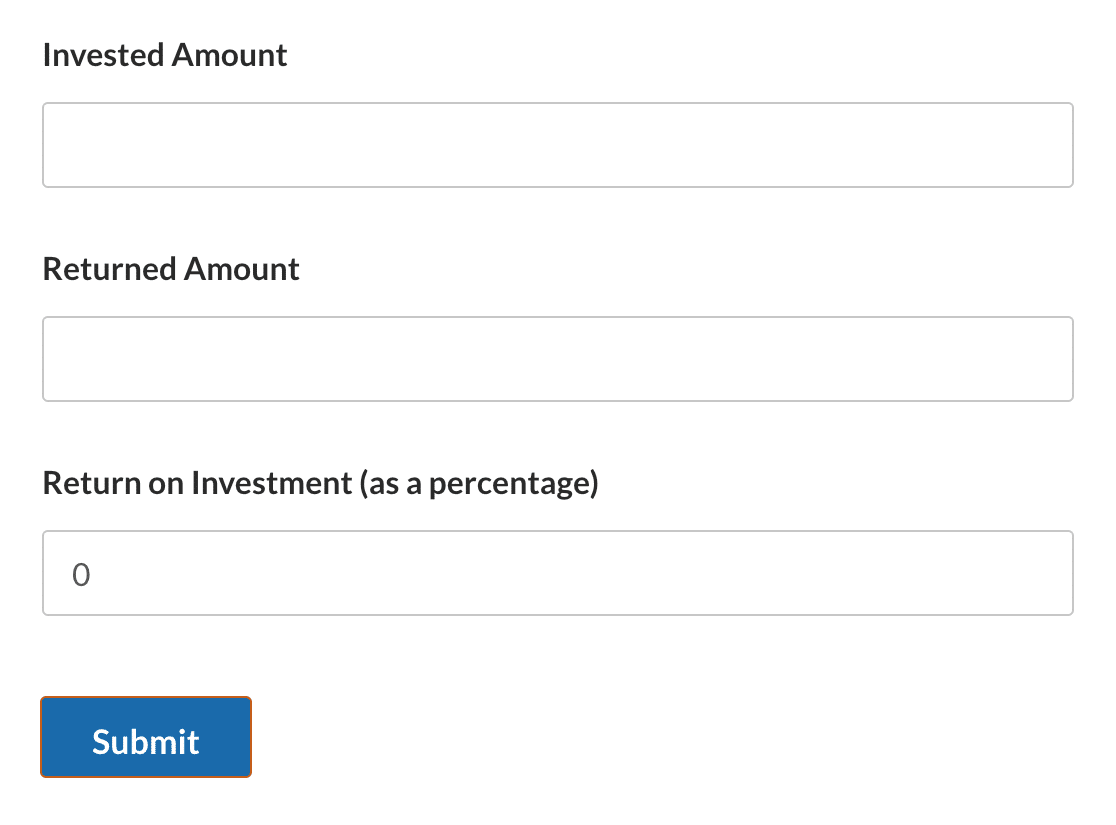Note: Check out our Return on Investment Calculator form template to use this form on your site.

##### Field Configurations
###### Field ID 1
• Field Type: Numbers
• Label: Investment Amount
• Field Variable: `\$F1`
###### Field ID 2
• Field Type: Numbers
• Label: Returned Amount
• Field Variable: `\$F2`
###### Field ID 3
• Field Type: Numbers
• Label: Return on Investment (as a percentage)
• Field Variable: `\$F3`
• Formula Overview: Determines the difference between the returned amount and the initial investment. It then divides this difference by the initial investment to find the proportionate increase or decrease. Multiplying by 100 converts this proportion into a percentage, which represents the Return on Investment (ROI).
• Formula:
`((\$F2 - \$F1) / \$F1) * 100`

#### Life Insurance Calculator

Determine the amount of insurance coverage needed based on age, income, dependents, and liabilities.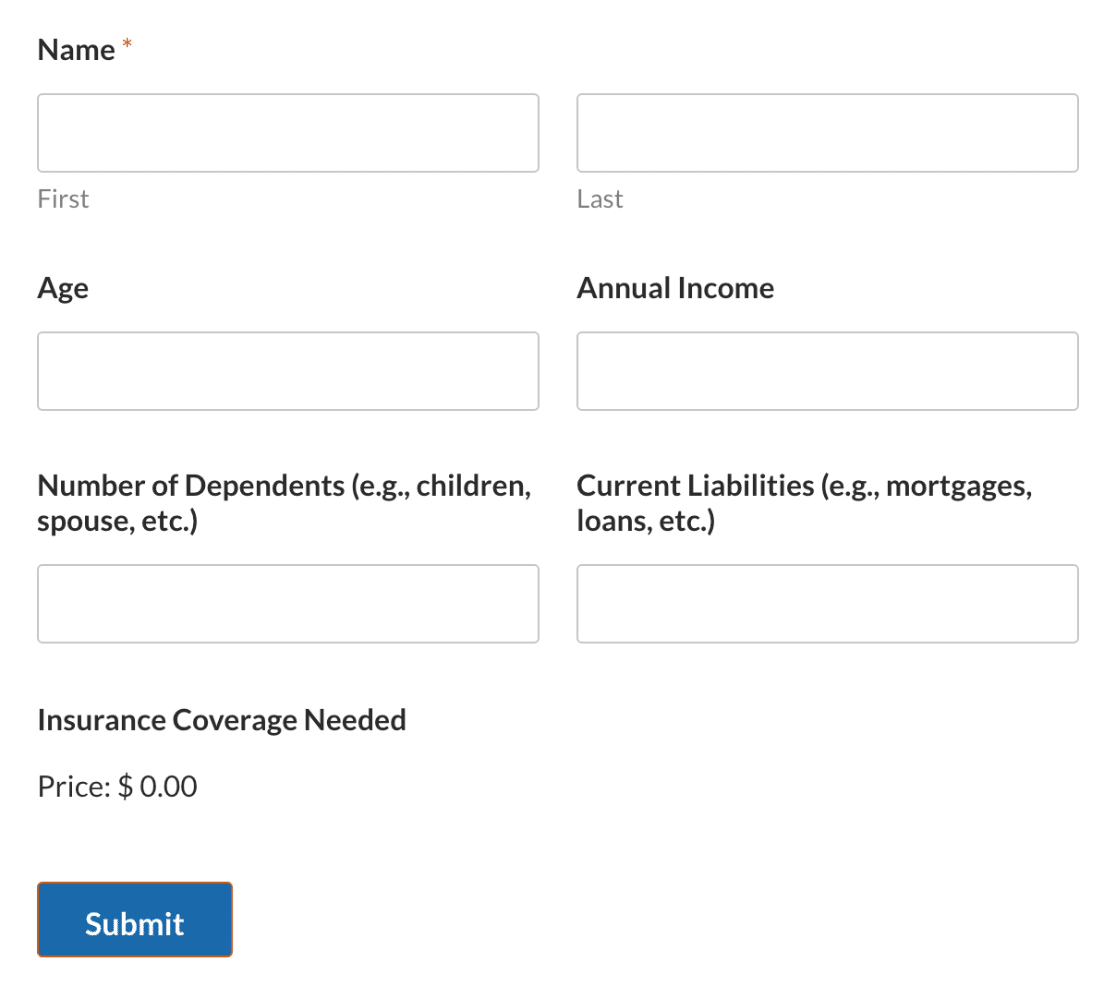Note: Check out our Life Insurance Calculator form template to use this form on your site.

##### Field Configurations
###### Field ID 2
• Field Type: Numbers
• Label: Age
• Field Variable: `\$F2`
###### Field ID 3
• Field Type: Numbers
• Label: Annual Income
• Field Variable: `\$F3`
###### Field ID 4
• Field Type: Numbers
• Label: Number of Dependents (e.g., children, spouse, etc.)
• Field Variable: `\$F4`
###### Field ID 5
• Field Type: Numbers
• Label: Current Liabilities (e.g., mortgages, loans, etc.)
• Field Variable: `\$F5`
###### Field ID 6
• Field Type: Single Item
• Label: Insurance Coverage Needed
• Field Variable: `\$F6`
• Formula Overview: Recommends insurance coverage by multiplying the annual income by 10 for individuals under 40, by 7 for those aged between 40 and 59, and by 5 for those 60 and above. Current liabilities are added to this result, and a sum based on the number of dependents is also factored in.
• Formula:
```if ( \$F2 < 40 ):
( \$F3  * 10)  +  \$F5 +  (  \$F4  *  \$F3  )
elseif( \$F2 >= 40 && \$F2 < 60 ):
( \$F3  * 7 )  +  \$F5  +  (  \$F4  *  \$F3 )
else:
(  \$F3  * 5 )  +   \$F5
endif;```

#### Stripe Fee Calculator

Calculate the Stripe fee based on the total amount selected from the available items in the order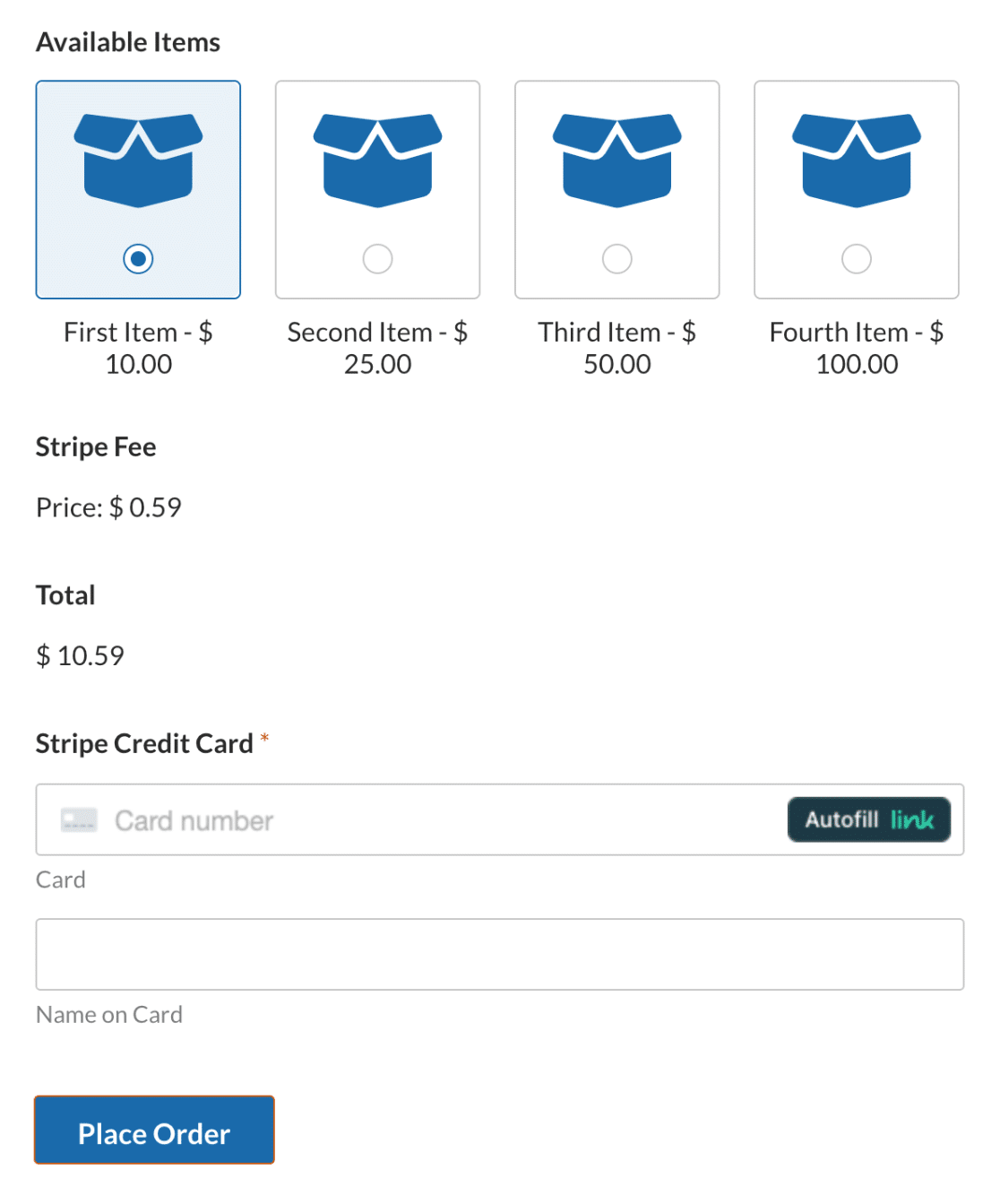Note: Check out our Stripe Fee Calculator form template to use this form on your site.

##### Field Configurations
###### Field ID 5
• Field Type: Multiple Items
• Label: Available Items
• Field Variable: `\$F5`
###### Field ID 6
• Field Type: Single Item
• Label: Stripe Fee
• Field Variable: `\$F6`
• Formula Overview: Calculates the Stripe fee based on the total amount selected from the available items. The Stripe fee is typically 2.9% of the transaction amount plus a fixed fee of \$0.30.
• Formula:
`(\$F5_amount * 0.029) + 0.30`

#### Lease Calculator

Calculate the monthly lease payment for an asset, considering the principal amount, down payment, interest rate, and lease duration.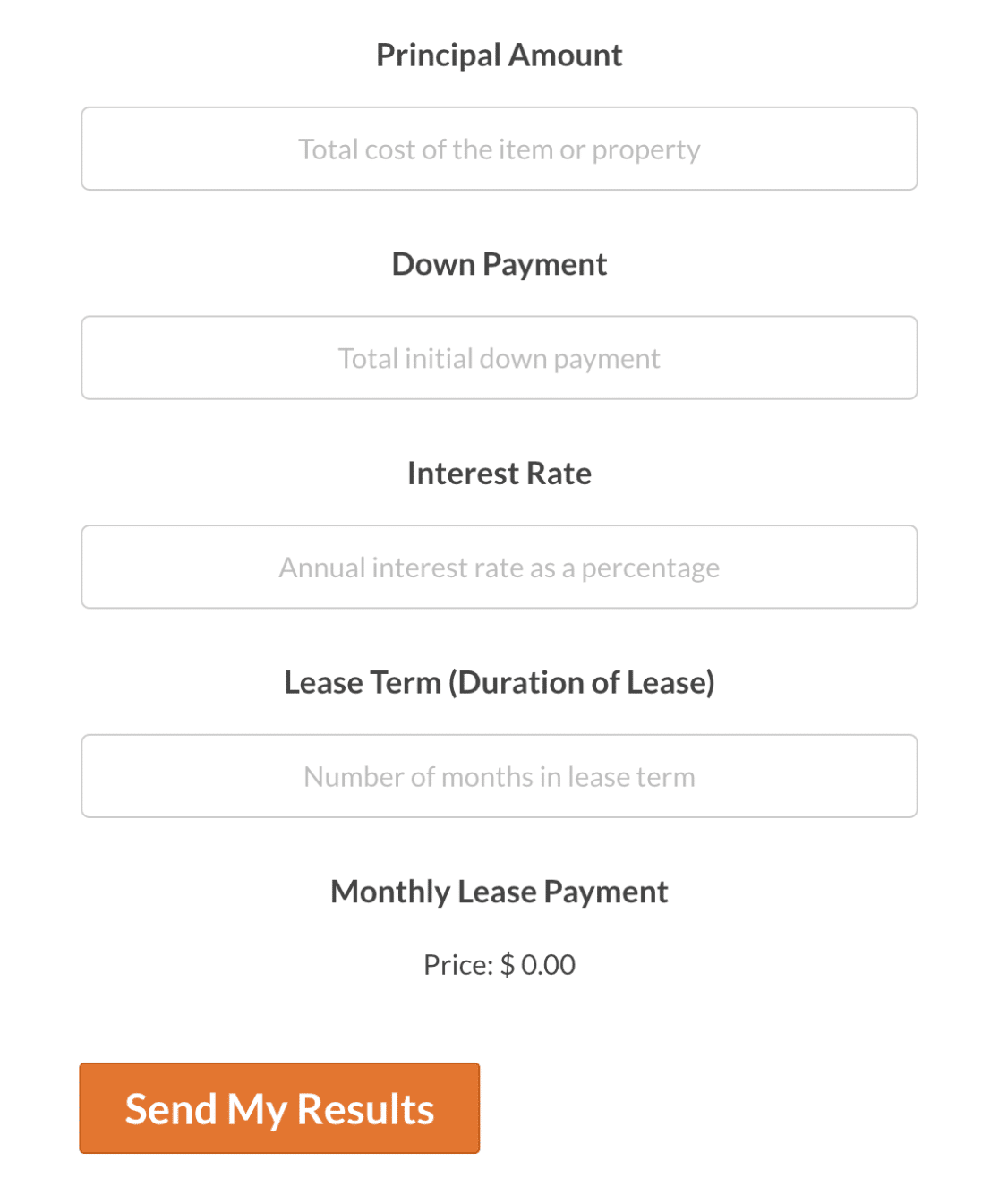Note: Check out our Lease Calculator form template to use this form on your site.

##### Field Configurations
###### Field ID 1
• Field Type: Numbers
• Label: Principal Amount
• Placeholder Text: Total cost of the item or property
• Field Variable: `\$F1`
###### Field ID 2
• Field Type: Numbers
• Label: Down Payment
• Placeholder Text: Total initial down payment
• Field Variable: `\$F2`
###### Field ID 3
• Field Type: Numbers
• Label: Interest Rate
• Placeholder Text: Annual interest rate as a percentage
• Field Variable: `\$F3`
###### Field ID 4
• Field Type: Numbers
• Label: Lease Term (Duration of Lease)
• Placeholder Text: Number of months in lease term
• Field Variable: `\$F4`
###### Field ID 5
• Field Type: Single Item
• Label: Monthly Lease Payment
• Field Variable: `\$F5`
• Formula Overview: Calculates the monthly lease payment by first subtracting the down payment from the principal amount. This amount is then divided by the lease term to distribute the cost evenly across the months. Additionally, the annual interest rate is converted to a monthly rate by dividing by 12, and then to a decimal form by dividing by 100. This monthly interest is applied to the principal minus down payment.
• Formula:
`(\$F1 - \$F2) / \$F4 + ((\$F1 - \$F2) * (\$F3 / 12 / 100))`

#### Dynamic Pricing Calculator

Create a payment form with dynamic pricing that adjusts the total cost based on the current date.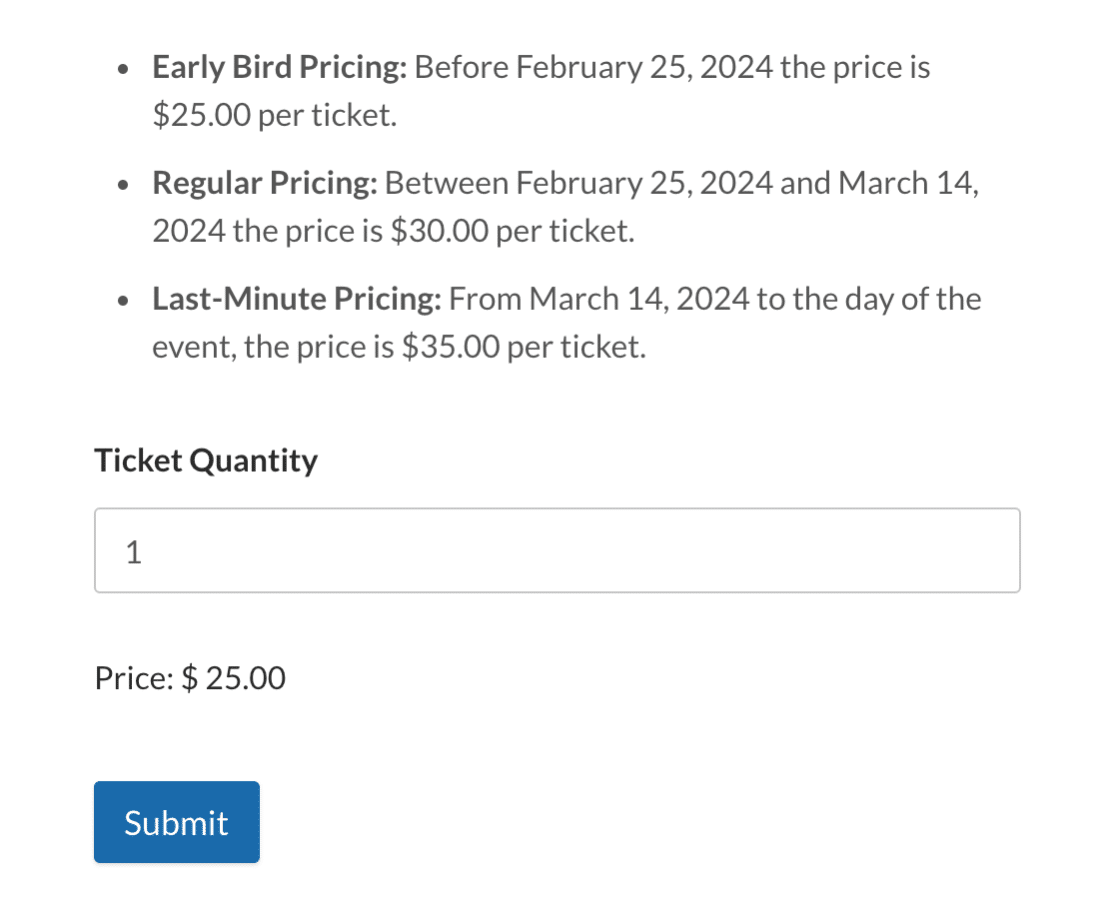Note: Check out our Dynamic Pricing Calculator form template to use this form on your site.

##### Field Configurations
###### Field ID 6
• Field Type: Numbers
• Label: Ticket Quantity
• Field Variable: `\$F6`
###### Field ID 7
• Field Type: Single Item
• Label: Hidden
• Field Variable: `\$F7`
• Formula Overview: Adjusts the ticket price based on the current date. The price per ticket changes based on whether the date is before February 25, 2024, between February 25 and March 14, 2024, or after March 14, 2024.
• Formula:
```if (now('d/m/Y') < "25/02/2024"):
\$F6 * 25.00
elseif (now('d/m/Y') >= "25/02/2024" && now('d/m/Y') < "14/03/2024"):
\$F6 * 30.00
else:
\$F6 * 35.00
endif; ```

Note: The dates in the formula are examples and may need updating. Be sure to modify them for your specific event or form requirements. For more info on using date/time functions in your calculations, see our guide to building formulas with the Calculations addon.

In this section, we’ll dive deeper into some more advanced formulas for the Calculations addon. These examples are ideal for those who are familiar with basic form calculations and want to try out more complex solutions.

#### Pay Raise Percent Calculator

Determine the increase in salary, based on the comparison between the current annual salary and the proposed new annual salary.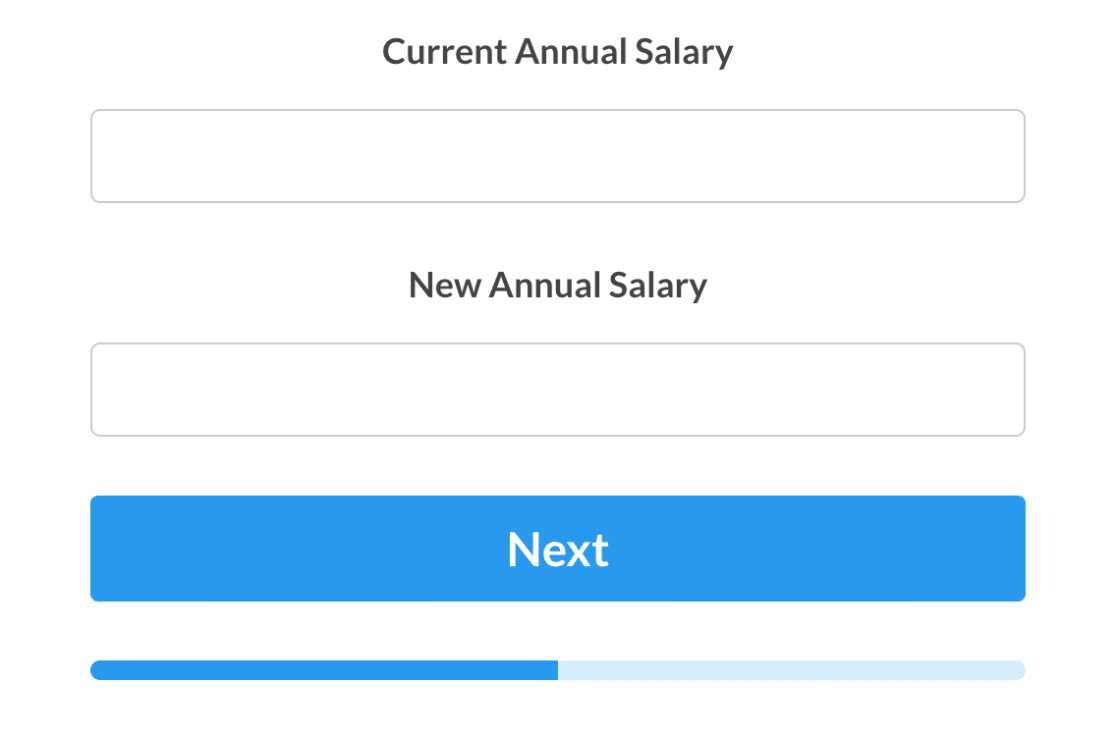Note: Check out our Pay Raise Percent Calculator form template to use this form on your site.

##### Field Configurations
###### Field ID 1
• Field Type: Numbers
• Label: Current Annual Salary
• Field Variable: `\$F1`
###### Field ID 2
• Field Type: Numbers
• Label: New Annual Salary
• Field Variable: `\$F2`
###### Field ID 3
• Field Type: Single Line Text
• Label: Pay Raise Percentage
• Input Mask: `alias:percentage`
• Field Variable: `\$F3`
• Formula Overview: Calculates the percentage difference between the new annual salary and the current annual salary, indicating the percentage increase.
• Formula:
`((\$F2 - \$F1) / \$F1) * 100`

Note: For information on creating aliases and input masks, check out our guide to using custom input masks.

###### Field ID 4
• Field Type: Single Line Text
• Label: Absolute Salary Increase
• Input Mask: `alias:currency`
• Field Variable: `\$F4`
• Formula Overview: Calculates the absolute difference between the new annual salary and the current annual salary, showing the total increase.
• Formula:
`( \$F2 - \$F1 )`
###### Field ID 5
• Field Type: Single Line Text
• Label: Monthly Increase
• Input Mask: `alias:currency`
• Field Variable: `\$F5`
• Formula Overview: Divides the absolute salary increase by 12 to calculate the average monthly increase in the salary
• Formula:
`( \$F4 / 12 )`
###### Field ID 6
• Field Type: Single Line Text
• Label: Weekly Increase
• Input Mask: `alias:currency`
• Field Variable: `\$F6`
• Formula Overview: Divides the absolute salary increase by 52 to determine the average weekly increment in the salary.
• Formula:
`( \$F4 / 52 )`
###### Field ID 7
• Field Type: Single Line Text
• Label: Daily Increase (assuming 5 working days a week)
• Input Mask: `alias:currency`
• Field Variable: `\$F7`
• Formula Overview: Finds the average daily salary increase by dividing the weekly increase by 5, assuming a typical 5-day working week.
• Formula:
`( \$F6  / 5 )`

#### Shipping Cost Calculator

Determine the estimated shipping cost based on the selected destination, package weight, and package dimensions.Note: Check out our Shipping Cost Calculator form template to use this form on your site.

##### Field Configurations
###### Field ID 1
• Field Type: Dropdown
• Label: Destination
• Choices: Local, Domestic, and International
• Field Variable: `\$F1`
###### Field ID 2
• Field Type: Numbers
• Label: Package Weight (lbs)
• Field Variable: `\$F2`
###### Field ID 3
• Field Type: Numbers
• Label: Length (cm)
• Field Variable: `\$F3`
###### Field ID 4
• Field Type: Numbers
• Label: Width (cm)
• Field Variable: `\$F4`
###### Field ID 5
• Field Type: Numbers
• Label: Height (cm)
• Field Variable: `\$F5`
###### Field ID 6
• Field Type: Hidden
• Field Variable: `\$F6`
• Formula Overview: Checks if the volume (Length * Width * Height) is greater than 1000 centimeters. If true, it adds \$5.00 to the shipping cost.
• Formula:
```if  (  \$F3  *  \$F4  *  \$F5 > 1000 ):
5
else:
0
endif;```
###### Field ID 7
• Field Type: Single Item
• Label: Estimated Shipping Cost (USD)
• Field Variable: `\$F7`
• Formula Overview: Shipping cost is calculated based on the selected destination and a weight multiplier. For Local destinations, the cost starts at \$5, for Domestic it’s \$10, and International it’s \$20. Twice the value of the package weight is then added to this cost. Finally, the amount from \$F6 is also added to determine the total shipping cost.
• Formula:
```if ( \$F1 == 'Local' ):
5 + (\$F2 * 2) +  \$F6
elseif ( \$F1 == 'Domestic' ):
10 + (\$F2 * 2) +  \$F6
else:
20 + (\$F2 * 2) +  \$F6
endif; ```

#### Length Conversion Calculator

Convert between different measurement units, including millimeters, centimeters, meters, kilometers, inches, feet, yards, and miles.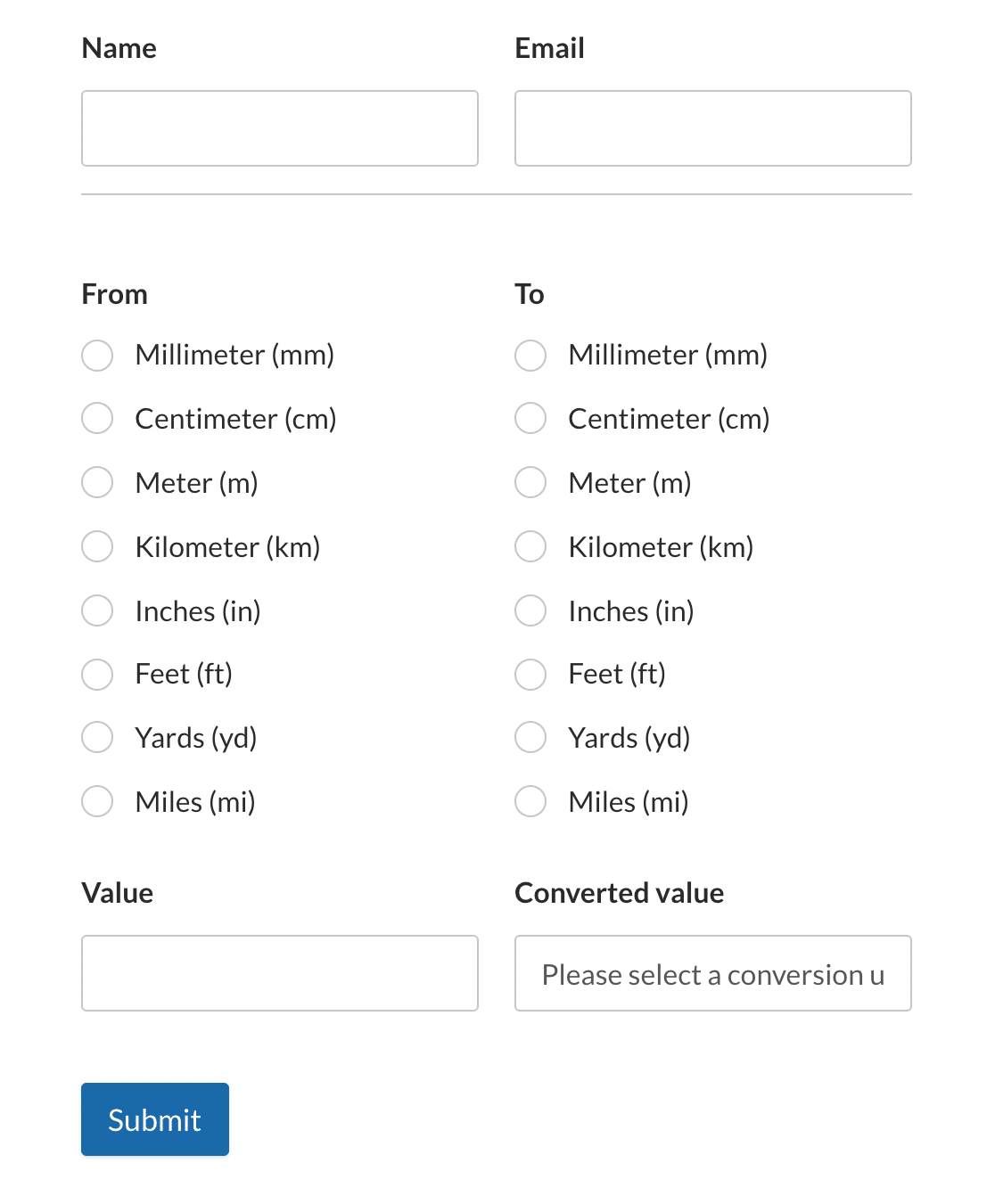Note: Check out our Length Conversion Calculator form template to use this form on your site.

##### Field Configurations
###### Field ID 6
• Field Type: Multiple Choice
• Label: From
• Choices: Millimeter (mm), Centimeter (cm), Meter (m), Kilometer (km), Inches (in), Feet (ft), Yards (yd), and Miles (mi)
• Field Variable: `\$F6`
###### Field ID 7
• Field Type: Single Line Text
• Label: Value
• Field Variable: `\$F7`
###### Field ID 8
• Field Type: Multiple Choice
• Label: To
• Choices: Millimeter (mm), Centimeter (cm), Meter (m), Kilometer (km), Inches (in), Feet (ft), Yards (yd), and Miles (mi)
• Field Variable: `\$F8`
###### Field ID 9
• Field Type: Hidden
• Label: Converted value
• Field Variable: `\$F9`
• Formula Overview: Checks if a conversion unit has been selected, then concatenates the initial value, the unit from which we’re converting, the converted value, and the unit to which we’re converting.
• Formula:
```if (\$F12== 0):
"Please select a conversion unit from above"
else:
concat(\$F7, " ", \$F6, " = ", \$F13, " ", \$F8)
endif;```
###### Field ID 11
• Field Type: Hidden
• Label: Meter conversion
• Field Variable: `\$F11`
• Formula Overview: Converts values from different units (mm, cm, km, inches, feet, yards, miles) to meters.
• Formula:
```if (\$F6 == "Millimeter (mm)"):
\$F7 / 1000
elseif (\$F6 == "Centimeter (cm)"):
\$F7 / 100
elseif (\$F6 == "Meter (m)"):
\$F7
elseif (\$F6 == "Kilometer (km)"):
\$F7 * 1000
elseif (\$F6 == "Inches (in)"):
\$F7 / 39.3701
elseif (\$F6 == "Feet (ft)"):
\$F7 / 3.28084
elseif (\$F6 == "Yards (yd)"):
\$F7 / 1.09361
elseif (\$F6 == "Miles (mi)"):
\$F7 / 0.000621371
else:
0
endif;```
###### Field ID 12
• Field Type: Hidden
• Label: Calculation
• Field Variable: `\$F12`
• Formula Overview: Converts values from meters to different units (mm, cm, km, inches, feet, yards, miles).
• Formula:
```if (\$F8 == "Millimeter (mm)"):
\$F11 * 1000
elseif (\$F8 == "Centimeter (cm)"):
\$F11 * 100
elseif (\$F8 == "Meter (m)"):
\$F11
elseif (\$F8 == "Kilometer (km)"):
\$F11 / 1000
elseif (\$F8 == "Inches (in)"):
\$F11 * 39.3701
elseif (\$F8 == "Feet (ft)"):
\$F11 * 3.28084
elseif (\$F8 == "Yards (yd)"):
\$F11 * 1.09361
elseif (\$F8 == "Miles (mi)"):
\$F11 * 0.000621371
else:
0
endif;```
###### Field ID 13
• Field Type: Hidden
• Label: Dynamic rounding
• Field Variable: `\$F13`
• Formula Overview: Dynamically rounds the value based on its size. If the absolute value is greater than or equal to 1, it rounds to 2 decimal places. If the value is between 0.01 and 1, it rounds to 4 decimal places. Otherwise, it rounds to 6 decimal places.
• Formula:
```if (abs(\$F12) >= 1):
round(\$F12, 2)
elseif (abs(\$F12) < 1 && abs(\$F12) >= 0.01):
round(\$F12, 4)
else:
round(\$F12, 6)
endif;```

Calculate mortgage payments based on the entered home value, down payment, interest rate, and mortgage period. Users input their relevant financial details and the form provides monthly and annual payment amounts, total interest paid, and the overall mortgage payment.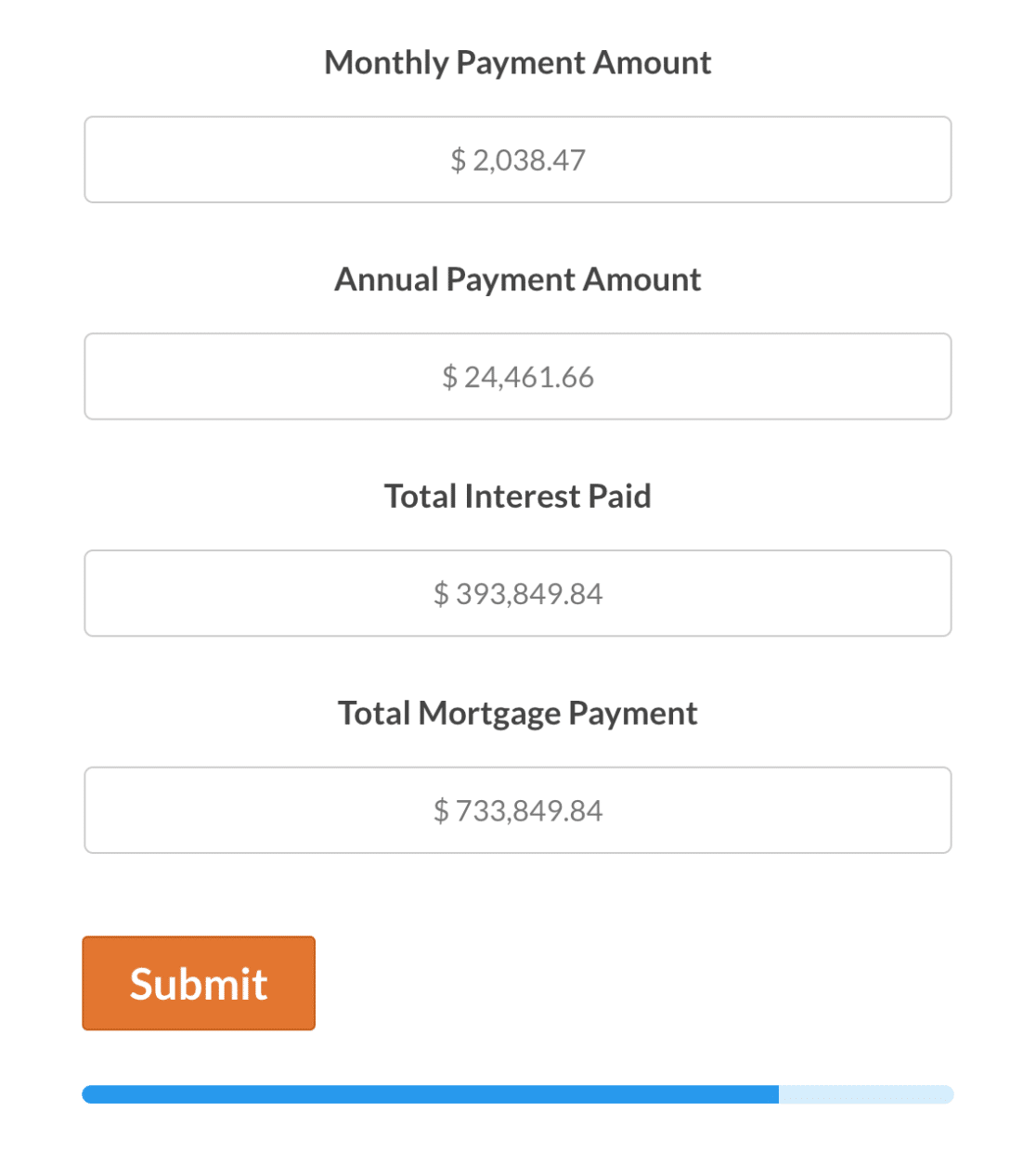Note: Check out our Mortgage Lead Generator form template to use this form on your site.

##### Field Configurations
###### Field ID 8
• Field Type: Single Item
• Label: Home Value
• Item Type: User Defined
• Field Variable: `\$F8`
###### Field ID 9
• Field Type: Single Item
• Label: Down Payment
• Item Type: User Defined
• Field Variable: `\$F9`
###### Field ID 16
• Field Type: Number Slider
• Label: Interest Rate
• Field Variable: `\$F16`
###### Field ID 17
• Field Type: Number Slider
• Label: Mortgage Period
• Field Variable: `\$F17`
###### Field ID 11
• Field Type: Single Line Text
• Label: Monthly Payment Amount
• Field Variable: `\$F11`
• Formula Overview: Calculates the monthly mortgage payment based on home value, down payment, interest rate, and mortgage period.
• Formula:
`format_amount(round(max(0, (\$F8_amount-\$F9_amount)* ((\$F16/12/100) * pow(1 + (\$F16/12/100),\$F17*12)) / (pow(1 + (\$F16/12/100),\$F17*12) - 1)), 2), 1)`
###### Field ID 12
• Field Type: Single Line Text
• Label: Annual Payment Amount
• Field Variable: `\$F12`
• Formula Overview: Calculates the annual mortgage payment based on home value, down payment, interest rate, and mortgage period.
• Formula:
`format_amount(round(max(0, 12 * ((\$F8_amount-\$F9_amount)* ((\$F16/12/100) * pow(1 + (\$F16/12/100), \$F17*12)) / (pow(1 + (\$F16/12/100), \$F17*12) - 1))), 2), 1)`
###### Field ID 13
• Field Type: Single Line Text
• Label: Total Interest Paid
• Field Variable: `\$F13`
• Formula Overview: Calculates the total interest paid over the life of the mortgage based on home value, down payment, interest rate, and mortgage period.
• Formula:
`format_amount(round((max(0, (\$F8_amount-\$F9_amount)* ((\$F16/12/100) * pow(1 + (\$F16/12/100), \$F17*12)) / (pow(1 + (\$F16/12/100), \$F17*12) - 1) * (\$F17*12)) - (\$F8_amount-\$F9_amount)), 2), 1)`
###### Field ID 14
• Field Type: Single Line Text
• Label: Total Mortgage Payment
• Field Variable: `\$F14`
• Formula Overview: Calculates the total mortgage payment, including principal and interest, based on home value, down payment, interest rate, and mortgage period.
• Formula:
`format_amount(round(max(0, (\$F8_amount-\$F9_amount)* ((\$F16/12/100) * pow(1 + (\$F16/12/100), \$F17*12)) / (pow(1 + (\$F16/12/100), \$F17*12) - 1) * (\$F17*12)), 2), 1)`

That’s it! Now you’ve seen multiple example formulas to help you get started with the Calculations addon.

Next, interested in offering discounts in your WordPress payment forms? Check out our guide to using the Coupons addon for all the details.